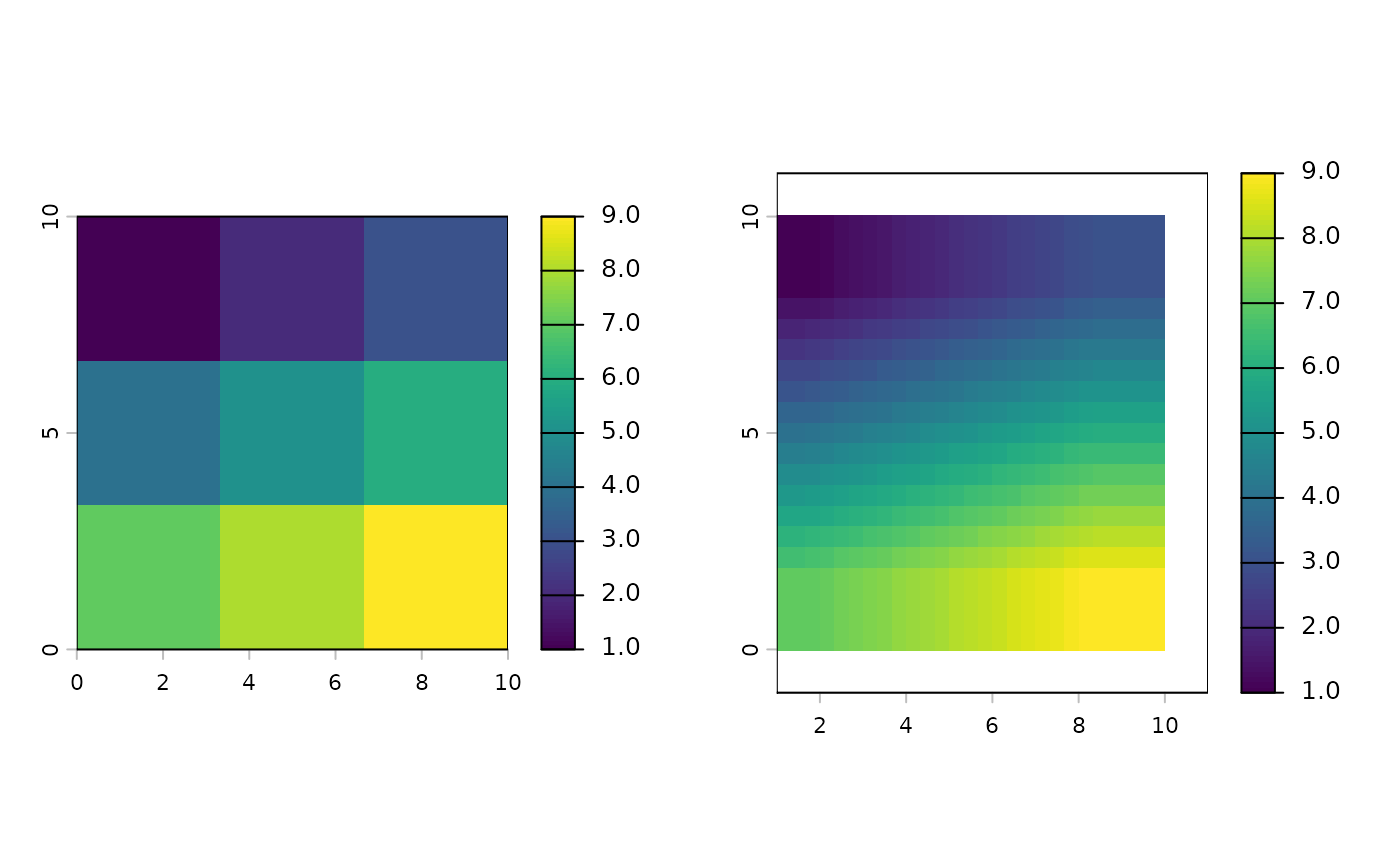resample transfers values between SpatRaster objects that do not align (have a different origin and/or resolution). See project to change the coordinate reference system (crs).

If the origin and extent of the input and output are the same, you should consider using these other functions instead: aggregate, disagg, extend or crop.

# S4 method for SpatRaster,SpatRaster
resample(x, y, method, threads=FALSE, filename="", ...)

## Arguments

x

SpatRaster to be resampled

y

SpatRaster with the geometry that x should be resampled to

method

character. Method used for estimating the new cell values. One of:

near: nearest neighbor. This method is fast, and it can be the preferred method if the cell values represent classes. It is not a good choice for continuous values. This is used by default if the first layer of x is categorical.

bilinear: bilinear interpolation. This is the default if the first layer of x is numeric (not categorical).

cubic: cubic interpolation.

cubicspline: cubic spline interpolation.

lanczos: Lanczos windowed sinc resampling.

sum: the weighted sum of all non-NA contributing grid cells.

min, q1, med, q3, max, average, mode, rms: the minimum, first quartile, median, third quartile, maximum, mean, mode, or root-mean-square value of all non-NA contributing grid cells.

logical. If TRUE multiple threads are used (faster for large files)

filename

character. Output filename

...

additional arguments for writing files as in writeRaster

## Value

SpatRaster

aggregate, disagg, crop, project,

## Examples


r <- rast(nrows=3, ncols=3, xmin=0, xmax=10, ymin=0, ymax=10)
values(r) <- 1:ncell(r)
s <- rast(nrows=25, ncols=30, xmin=1, xmax=11, ymin=-1, ymax=11)
x <- resample(r, s, method="bilinear")